# Atomic StructurePage 2

#### WATCH ALL SLIDES

These obviously have the same solutions separately as our original particle in an infinite square well, and corresponding energies:

Slide 7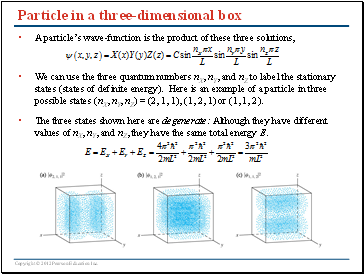Particle in a three-dimensional box

A particle’s wave-function is the product of these three solutions,

We can use the three quantum numbers nX, nY, and nZ to label the stationary states (states of definite energy). Here is an example of a particle in three possible states (nX, nY, nZ) = (2, 1, 1), (1, 2, 1) or (1, 1, 2).

The three states shown here are degenerate: Although they have different values of nX, nY, and nZ, they have the same total energy E.

Slide 8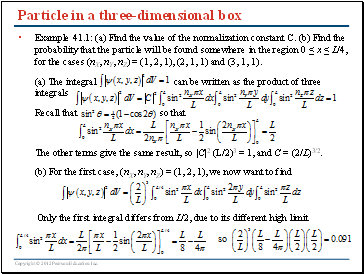Particle in a three-dimensional box

Example 41.1: (a) Find the value of the normalization constant C. (b) Find the probability that the particle will be found somewhere in the region 0 ≤ x ≤ L/4, for the cases (nX, nY, nZ) = (1, 2, 1), (2, 1, 1) and (3, 1, 1).

(a) The integral can be written as the product of three integrals

Recall that so that

The other terms give the same result, so |C|2 (L/2)3 = 1, and C = (2/L)3/2.

(b) For the first case, (nX, nY, nZ) = (1, 2, 1), we now want to find

Only the first integral differs from L/2, due to its different high limit

Slide 9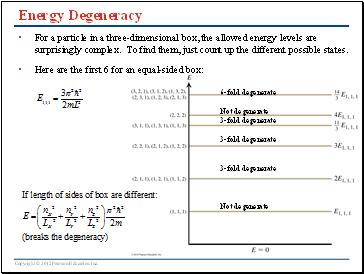## Energy Degeneracy

For a particle in a three-dimensional box, the allowed energy levels are surprisingly complex. To find them, just count up the different possible states.

Here are the first 6 for an equal-sided box:

6-fold degenerate

Not degenerate

3-fold degenerate

3-fold degenerate

3-fold degenerate

Not degenerate

Slide 10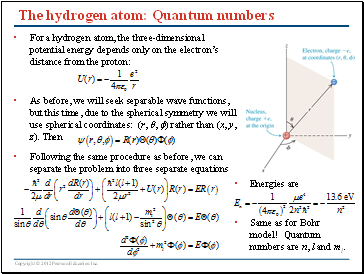## The hydrogen atom: Quantum numbers

Energies are

Same as for Bohr model! Quantum numbers are n, l and ml.

For a hydrogen atom, the three-dimensional potential energy depends only on the electron’s distance from the proton:

As before, we will seek separable wave functions, but this time, due to the spherical symmetry we will use spherical coordinates: (r, , ) rather than (x, y, z). Then

Following the same procedure as before, we can separate the problem into three separate equations

Slide 11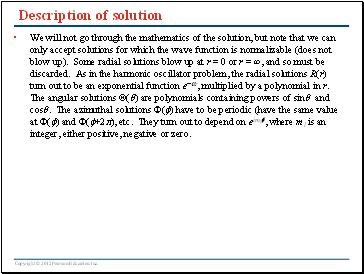## Description of solution

We will not go through the mathematics of the solution, but note that we can only accept solutions for which the wave function is normalizable (does not blow up). Some radial solutions blow up at r = 0 or r = ∞, and so must be discarded. As in the harmonic oscillator problem, the radial solutions R(r) turn out to be an exponential function e-ar, multiplied by a polynomial in r. The angular solutions Q(q) are polynomials containing powers of sinq and cosq. The azimuthal solutions F(f) have to be periodic (have the same value at F(f) and F(f+2p), etc. They turn out to depend on eimlf, where ml is an integer, either positive, negative or zero.

Go to page:
1  2  3  4  5  6